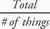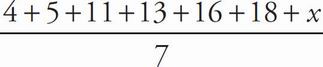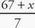# SAT Math Multiple Choice Question 134: Answer and Explanation

### Test Information

Question: 134

14. John Croxley, the mayor of Black Rock, NY, is counting the number of restaurants that have opened in his town per month for the last seven months. He compiles the seven numbers into Set F, which contains the elements 4, 5, 11, 13, 16, 18, and x. If both the median and average (arithmetic mean) of Set F equal 11, what must be the value of x, the unknown number of restaurants that opened in Mayor Croxley's town last month?

• A. 9
• B. 10
• C. 11
• D. 12

B The formula for determining an average can be expressed by Average =. Plug the values provided into the equation as follows: 11 =, or 11 =. Multiply both sides by 7 to get 77 = 67 + x. Subtract 67 from both sides to get x = 10, which is (B).